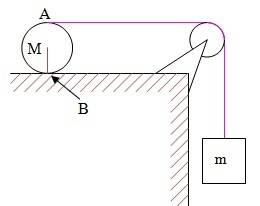# Mass pulling another, rotating, mass

## Homework Statement[/B]
The cylinder rolls without friction. what's m's acceleration, M's angular acceleration and the tension.

## Homework Equations

Shteiner's theorem: ##I_c=I_{c.o.m.}+Mr^2##
Torque and angular acceleration: ##M=I\alpha##
Moment of inertia of a solid cylinder: ##I_{cen}=\frac{mr^2}{2}##

## The Attempt at a Solution

I denote the center variables with c
Kinematics (or geometry? which discipline is suitable for this relation):
$$x_c=\omega r~~\rightarrow~~\dot x_c=\alpha r~~\rightarrow~~\alpha=\frac{\dot x_c}{r}$$
$$\left\{ \begin{array}{l} mg-T=m\dot x_A~~\rightarrow~~T=m(g-\dot x_A)=m(g-2\dot x_c) \\ 2r\cdot T=I_B\frac{\dot x_c}{r} \end{array} \right.$$
$$\rightarrow~\dot x_c=\frac{2r^2mg}{I_B+4mr^2}=\frac{2r^2mg}{I_c+Mr^2+4mr^2}=\frac{2r^2mg}{\frac{Mr^2}{2}+(M+4m)r^2}=\frac{4mg}{3M+8m}$$